```SELECTED PRINCIPLES: REDOX REACTIONS (2)
Reducing agents are substances which cause reduction, and oxidizing
agents are substances which cause oxidation. This uninspiring sentence,
though correct, obscures the fact that the terms 'reducing agent' and
'oxidizing agent' are relative, as the two following examples of redox
reactions should illuminate.
First, magnesium and dihydrogen are both termed reducing agents: but
magnesium is the stronger one. Thus, in the following redox reaction,```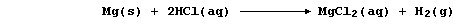```electrons are transferred from magnesium atoms to hydrogen ions because
magnesium is a stronger reductant (i.e., it is more easily oxidized);
the redox half equations are:```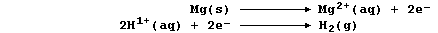`The aqueous chloride ions are spectators, so the net ionic equation is:`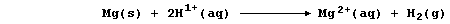```And second, both bromine and iodine are termed oxidizing agents: but
bromine is the stronger one. Thus, in the following redox reaction,```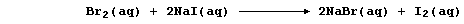```electrons are transferred from iodide ions to bromine atoms because
bromine is a stronger oxidant (i.e., it is more easily reduced); the
redox half equations are:````The aqueous sodium ions are spectators, so the net ionic equation is:`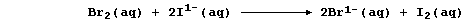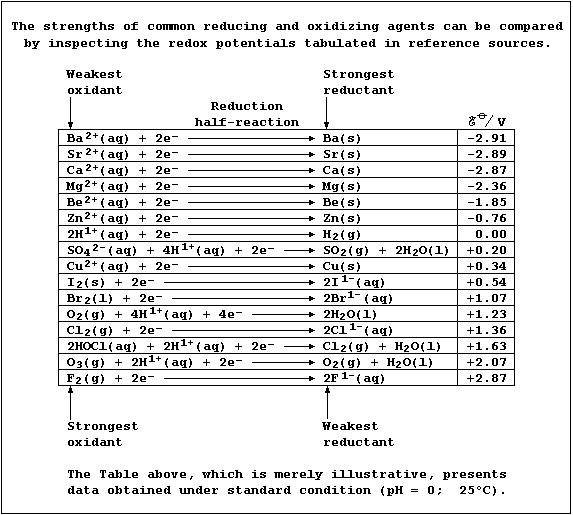```1.  The elements in Group 17 are collectively known as the halogens.
All isotopes of astatine are radioactive; its longest-lived isotope is
astatine-210, which has half-life of 8.3 hours: therefore, it is both
difficult and expensive to study astatine in a laboratory.
(a) Complete this first Table, by predicting properties of diastatine.```
 ` Property ` ` F2 ` ` Cl2 ` ` Br2 ` ` I2 ` ` As2 ` ` Melting point / įC` ` -223` ` -102` ` -7` ` 114` ` ` ` Boiling point / įC` ` -188` ` -35` ` 59` ` 183` ` ` ``` Appearance at r.t.p. (25įC; 100 kPa)``` ``` pale yellow gas``` ``` yellow- green gas``` ``` red- brown liquid ``` ``` dark grey solid ``` ` ` ` Bond energy / kJ mol-Ļ` ` 151` ` 243` ` 193` ` 151` ` `
```                                                                    
(b) Complete this second Table, to show the radioactive decay of At-210.```
 ` Time / hours` ` 0.0` ` 8.3` ` 16.6` ` ` ` ` ` ` ` Number of half-lives elapsed ` ` 0 ` ` 1 ` ` 2 ` ` ` ` ` ` ` ` Mass of 210As / 10-9 g ` ` 96 ` ` 48 ` ` 24 ` ` ` ` ` ` `
```                                                                    
2.  Chlorine gas dissolves in water to give so-called 'chlorine water',
a rather loose term used to indicate the following equilibrium mixture:````The hydrochloric acid is completely dissociated, being a strong acid,`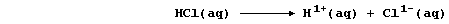`and the chloric(I) acid is partially dissociated, being a weak acid,`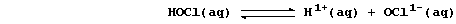```Aqueous hydrogen and chlorate(I) ions both diffuse into cells, across
semi-permeable membranes, where hydrogen ions can denature enzymes
and chlorate(I) ions act as biocides by oxidizing essential biological
molecules. Name two types of molecules, which are involved in growth,
reproduction, or respiration, which might be oxidized by chlorate(I)
ions. _________________________________________________________________

3.  Shown below is a flow diagram for the extraction of bromine from
sea water, where it occurs as bromide ions (their concentration is low;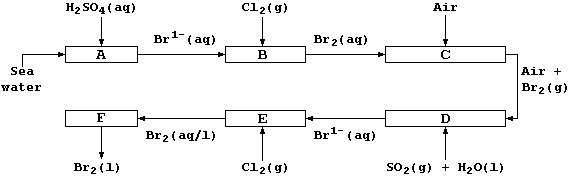```(a) Construct the net ionic equation for the reactions at Stages B and